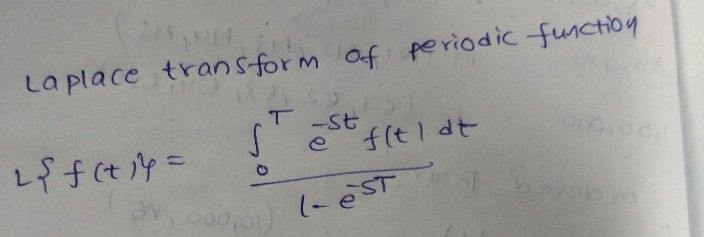Symbol
Problem$4.$ Find the Laplace Transform of the periodic function f whose graph is given below. $1$ $-1$ $\bar{2α}$ $4a$ $\vec{6a}$
Calculus
Search count: 106
SolutionQanda teacher - varshini